# Percentage TCS Questions for SSC CGL 2021

Validity: 9 Months
What you will get
Course Highlights
• Based on latest Pattern
• English Medium eBooks
SSC

## Percentage TCS Questions for SSC CGL 2021

Here we provide previous year questions and solutions of percentage asked by TCS in SSC CGL pre-exam in 2019. Each question is in Hindi/English, date-wise. These questions are important for upcoming exams of SSC CGL, CHSL, CPO, and various others.

Q1. If decreasing 120 by x% gives the same result as increasing 40 by x% then x% of 210 is what percent less than (x + 20)% of 180?

यदि 120 को x% कम करने से वही परिणाम आता है जो 40 को x% बढ़ाने से आता है, तो 210 का x% 180 के (x+20) % से कितना प्रतिशत कम है ?

SSC CGL 4 June 2019 (Morning)

(a) 331/3

(b) 18

(c) 16 2/3

(d) 20

Q2. The income of Raju is 20% more than his expenditure. If his income increases by 60% and his expenditure increases by 70% then by what percent does his savings increase or decrease?

राजू की आय उसके व्यय से 20% अधिक है | यदि उसकी आय 60% बढ़ जाती है और उसके व्यय में 70% की वृद्धि हो जाती है, तो उसकी बचत में कितने प्रतिशत की कमी या वृद्धि होगी ?

SSC CGL 4 June 2019 (Afternoon)

(a) It decreases by 10%/ यह 10% कम हो जाएगी

(b) It decreases by 2%/ यह 2% कम हो जायेगी

(c) It increases by 10%/ यह 10% बढ़ जाएगी

(d) It increases by 2%/ यह 2% बढ़ जाएगी

Q3. The income of A is 25% more than that of B and the income of C is 65% less than the sum of the incomes of A and B. Income of C is what percent less than the income of A?

A की आय B की आय से 25% अधिक है और C की आय A और B की आय के योग से 65% कम है | C की आय A की आय से कितना प्रतिशत कम है ?

SSC CGL 4 June 2019 (Evening)

(a) 28

(b) 32

(c) 35

(d) 37

Q4. The income of A is 50% more than that of B. If the income of A is increased by 40% and the income of B is increased by 90%, then the percentage increase in their combined income will be:

A की आय B की आय से 50% अधिक है | यदि A की आय 40% से बढ़ा दी जाए और B की आय 90% से बढ़ा दी जाए, तो उनकी संयुक्त आय में कितने प्रतिशत की वृद्धि होगी ?

SSC CGL 6 June 2019 (Morning)

(a)    64

(b)   55

(c)    60

(d)   70

Q5. When the price of an item was reduced by 20%,  then the sale increased by x%. If there is an increase of 60% in receipt of the revenue, then the value of x is :

जब किसी वस्तु की कीमत 20% कम कर दी गयी, तो इसकी बिक्री x% से बढ़ गयी | यदि आय की प्राप्ति में 60% की वृद्धि होती है, तो x का मान है :

SSC CGL 6 June 2019 (Afternoon)

(a) 120

(b) 96

(c) 100

(d) 80

Q6. A is 20% less than B and C is 30% more than D. If D is 25% less than A, then which of the following is true?

A, B से 20% कम है और C, D से 30% अधिक है | यदि D, A से 25% कम है, तो निम्न में से क्या सही है ?

SSC CGL 7 June 2019 (Morning)

(a) B = 0.39C

(b) C = 0.78B

(c) B = 0.78C

(d) C = 0.39B

Q7. The price of sugar has increased by 20%. A person wants to increase its expenditure by 8% only. By what percent should he decrease his consumption?

चीनी की कीमत 20% से बढ़ जाती है | एक व्यक्ति अपना व्यय केवल 8% बढ़ाना चाहता है | उसे अपनी खपत में कितने प्रतिशत की कमी करनी चाहिए ?

SSC CGL 10 June 2019 (Afternoon)

(a) 10%

(b) 11%

(c) 9%

(d) 12%

Q8. If 85% of a number is added to 75, then the result is the number itself. The number is:

यदि किसी संख्या के 85% को 75 में जोड़ा जाए, तो परिणामस्वरूप वह संख्या स्वयं प्राप्त होती है | वह संख्या है :

SSC CGL 13 June 2019 (Evening)

(a)500

(b)200

(c)300

(d)100

Q9. Sudha saves 15% of her income. If her expenditure increases by 20% and savings increases by 60% , then by what percent has her income increased?

सुधा अपनी आय का 15% बचत करती है | यदि उसका व्यय 20% से बढ़ जाता है तथा बचत में 60% की वृद्धि हो जाती है, तो उसकी आय में कितने प्रतिशत की वृद्धि हुई है ?

SSC CGL 6 June 2019 (Evening)

(a) 26

(b) 35

(c) 24

(d) 30

Q10. A is 20% less than B and C is 20% more than D. If D is 25% less than A , then which of the following is true? / B से 20% कम है और C, D से 20% अधिक है | यदि D, A से 25% कम है, तो निम्न में से कौन सा सही है ?

SSC CGL 7 June 2019 (Afternoon)

(a) B = 0.675C

(b) C = 0.72B

(c) B = 0.72C

(d) C = 0.675B

Q11. Surbhi spends 75% of her income. If her income increases by 20% and savings decreases by 1%, then the percentage increase in her expenditure is :

सुरभि अपनी आय का 75% खर्च करती है | यदि उसकी आय 20% से बढ़ जाए और उसकी बचत में 1% की कमी हो जाए, तो उसके व्यय में हुई प्रतिशत वृद्धि ज्ञात करें |

SSC CGL 7 June 2019 (Evening)

(a) 27

(b) 2.2

(c) 22

(d) 2.7

Q12. The income of A is 40 % more than that of B. A got a 25% rise in his income and B got a 40%  rise in his income, then the percentage increase in the combined incomes of A and B is :

A की आय B की आय से 40% अधिक है | A की आय 25% बढ़ गयी और B की आय 40% बढ़ गयी, तो A और B की संयुक्त आय में प्रतिशत वृद्धि ज्ञात करें |  SSC CGL 10 June 2019 (Morning)

(a) 31.25

(b) 34.5

(c) 28.25

(d) 24.5

Q13. The price of sugar has increased by 18%. A person wants to increase the expenditure by 12% only. By what percent, correct to one decimal place, should he decrease his consumption?

चीनी की कीमत 18% बढ़ जाती है | एक व्यक्ति अपना व्यय केवल 12% बढ़ाना चाहता है| उसे अपनी खपत में कितने प्रतिशत ( एक दशमलव स्थान तक सही ) की कमी करनी चाहिए ?

SSC CGL 10 June 2019 (Evening)

(a) 6%

(b) 5.1%

(c) 5.3%

(d) 5.6%

Q14. The price of sugar has increased by 22%. A person wants to increase his expenditure by 12% only. By what percent should he decrease his consumption, nearest to one decimal place?

चीनी की कीमत 22% बढ़ जाती है | एक व्यक्ति अपने व्यय में केवल 12% की वृद्धि करना चाहता है | उसे अपनी खपत में कितने प्रतिशत की कमी ( एक दशमलव स्थान तक सही ) करनी चाहिए ?

SSC CGL 11 June 2019 (Morning)

(a) 10%

(b) 7.8%

(c) 8.2%

(d) 8.6%

Q15. The price of sugar has increased by 17%. A person wants to increase his expenditure by 8% only. By what percent should he decrease his consumption, nearest to one decimal place?

चीनी की कीमत 17% से बढ़ जाती है | एक व्यक्ति अपना व्यय केवल 8% से बढ़ाना चाहता है | उसे अपनी खपत में कितने प्रतिशत ( एक दशमलव स्थान तक सही ) की कमी करनी चाहिए ?

SSC CGL 11 June 2019 (Afternoon)

(a) 7.9%

(b) 8.3%

(c) 8.1%

(d) 7.7%

Q16. The price of sugar is increased by 21%. A person wants to increase his expenditure by 12% only. By what percent should he decrease his consumption, nearest to one decimal place?

चीनी की कीमत 21% से बढ़ जाती है | एक व्यक्ति अपना व्यय केवल 12% बढ़ाना चाहता है | उसे अपनी खपत में कितने प्रतिशत ( एक दशमलव स्थान तक सही ) की कमी करनी चाहिए ? SSC CGL 11 June 2019 (Evening)

(a) 7.4%

(b) 7.2%

(c) 7.6%

(d) 7.8%

Q17. The price of sugar is increased by 17%. A person wants to increase his expenditure by 7% only. By what percentage is correct to one decimal place, should he reduce his consumption?

चीनी की कीमत 17% से बढ़ जाती है | एक व्यक्ति अपना व्यय केवल 7% बढ़ाना चाहता है | उसे अपनी खपत में कितने प्रतिशत ( एक दशमलव स्थान तक सही ) की कमी करनी चाहिए ? SSC CGL 12 June 2019 (Morning)

(a) 8.1%

(b) 8.3%

(c) 8.5%

(d) 8.7%

Q18. The price of sugar is increased by 24%. A person wants to increase his expenditure by 15% only. By what percentage, correct to one decimal place, should he reduce his consumption?

चीनी की कीमत 24% से बढ़ जाती है  | एक व्यक्ति अपना व्यय केवल 15% से बढ़ाना चाहता है | उसे अपनी खपत में कितने प्रतिशत ( एक दशमलव स्थान तक सही ) की कमी करनी चाहिए ? SSC CGL 12 June 2019 (Afternoon)

(a) 7.3

(b) 7.1

(c) 6.9

(d) 7.5

Q19. The price of sugar has increased by 24%. A person wants to increase his expenditure by 18% only. By approximately what percent should he decrease his consumption?

चीनी की कीमत 24% बढ़ जाती है | एक व्यक्ति अपना व्यय केवल 18% बढ़ाना चाहता है | उसे अपनी खपत में लगभग कितने प्रतिशत की कमी करनी चाहिए ? SSC CGL 12 June 2019 (Evening)

(a) 4.6%

(b) 5.1%

(c) 4.8%

(d) 5.3%

Q20. The price of petrol has increased by 28%. A person wants to increase his expenditure by 22% only. By approximately what percent should decrease his consumption?

पेट्रोल की कीमत 28% से बढ़ जाती है | एक व्यक्ति अपना व्यय केवल 22% से बढ़ाना चाहता है | उसे अपनी खपत में लगभग कितने प्रतिशत की कमी करनी चाहिए ?

SSC CGL 13 June 2019 (Morning)

(a)5.3%

(b)4.7%

(c)4.9%

(d)5.1%

Q21.The price of sugar has increased by 17%. A person wants to increase his expenditure by 5% only. By approximately what percent should he decrease his consumption?

चीनी की कीमत 17% से बढ़ जाती है | एक व्यक्ति अपना व्यय केवल 5% से बढ़ाना चाहता है | उसे अपनी खपत में लगभग कितने प्रतिशत की कमी करनी चाहिए ?

SSC CGL 13 June 2019 (Afternoon)

(a)10.3

(b)10.7

(c)10.9

(d)9.9

Here are the solutions to the above questions of Percentage asked by TCS in SSC CGL Tier 1.

Sol 1. (c)

According to the question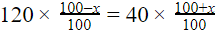300-3x = 100 + x

X = 50

50% of 210 = 105

(50+20)% of 180 = 126

Required percentage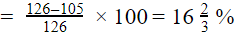Sol 2. (c)

Income : Expenditure Savings

60     :        50       →         10

After increase in Income and Expenditure.

96    :          85       →       11

Therefore, Net Increase in Savings = 1/10 × 100=10%

Sol 3. (d)

25% = 1/4

⇒ A : B = 5 : 4

65% = 13/20

⇒ C : A+B = 7 : 20

Balancing the ratio for A + B

A = 100, B = 80 and C = 63

Required % age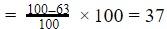Sol 4. (c)

50% = 1/2

A:B = 3:2

Let income of A = 300 unit and B = 200 unit

Total = 300+200 = 500 unit

Increased income of A =300× 140/100= 420

Increased income of B = 200× 190/100= 380

Total income of A and B = 420+380 = 800

Required % =  800-500 /500  × 100= 60%

Sol 5.  (c) 20% = 1/5

Let the original price = 50 unit

⇒ decreased price = 40 unit

Let the original sale = 100 unit

⇒ New sale = (100+x) unit

Initial  revenue = 50 x 100 units

⇒ Increased revenue = 40 ×  (100+x) units

According to the question

(50 x 100)× 160/100=  40 ×  (100+x)

⇒ 200 = 100 + x

⇒ x = 100

Sol 6. (b)

20% = 1/5 , 30% = 3/10 and 25% = 1/4

⇒ A:B = 4:5 and C:D = 13 : 10 and A:D = 4 : 3

Balancing the ratio for A and D

A:B:C:D = 40:50:39:30

Let

B = 50 unit and C = 39 unit

Now, 50 78100= 39

⇒ C is 0.78 of B .

Sol 7. (a)

We know that,

Price x Consumption = Expenditure

20% = 1/5 and 8% = 2/25

Initial : New

Price                                   5     :     6

Expenditure                       25   :    27

_______________

Consumption                 25/5   : 27/6

10   :   9

%age decrease in consumption = 10-9/10 ×  100 = 10% ans

Alternate :

Direct Trick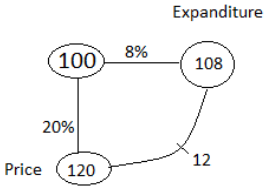Desired percentage = 12120x 100 = 10%

Sol 8. (a)

85% = 17/20

Let the original number = 20

According to the question

75 + 17 unit = 20 unit

1 unit = 25

Original number (20 unit) = 25 x 20 = 500

Sol 9. (a)

15% = 3/20

Let sudha’s income = 2000

⇒ Sudha’s saving = 300

⇒ Sudha’s expenditure = 2000-300 = 1700

Sudha’s increased saving = 300 x 160/100= 480

Sudha’s increased expenditure = 1700 x 120/100= 2040

Sudha’s increased income = 2040+480 = 2520

Desired percentage = 2520-2000/2000 × 100 = 26 %

Sol 10.(b)

20% = 1/5 and 25% = 1/4

A:B = 4:5 and C:D = 6 : 5 and A:D = 4 : 3

Balancing the ratio for A and D

A:B:C:D = 20:25:18:15

Let

B = 25 unit and C = 18 unit

Now, 25 × 72/100= 18

⇒ C is 0.72 of B .

Sol 11. (a)

75% = 3/4

Let the income of Surbhi = 400 unit

saving of Surbhi = 100 unit

So expenditure of Surbhi = 400-100 = 300 unit

Increased income of surbhi = 400× 220/100= 480 unit

Decreased saving of Surbhi = 100× 99/100= 99 unit

New expenditure of  surbhi = 480-99 = 381 unit

Desired %age =381-300/300 × 100 = 27 %

Sol 12. (a)

40% = 2/5

Let the income of B = 500

⇒ The income of A = 700

Total income of A and B = 1200

Increased income of A = 700 × 125/100= 875

Increased income of B = 500 × 140/100= 700

Total income of A and B = 875+700 = 1575

Desired %age=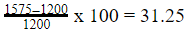Sol 13. (b)

We know that,

Price x Consumption = Expenditure

18% = 9/50 and 12% = 3/25

Initial : New

Price                    50     :    59

Expenditure         25    :    28

_______________

Consumption    25/50  :  28/59

59    :   56

%age decrease in consumption =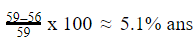Alternate :

Direct TrickDesired percentage = 6/118  x 100 ≈ 5.1%

Sol 14. (c)

We know that,

Price x Consumption = Expenditure

22% = 1150 and 12% = 325

Initial : New

Price                    50     :    61

Expenditure          25    :    28

_______________

Consumption    25/50  :  28/61

61    :   56

%age decrease in consumption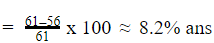Alternate :

Direct Trick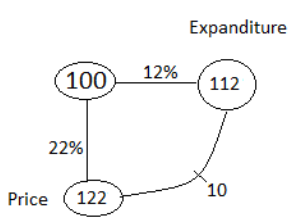Desired percentage = 10/122 ×  100 ≈ 8.2%

Sol 15. (d)

We know that,

Price x Consumption = Expenditure

17% = 17100 and 8% = 225

Initial : New

Price                   100   :   117

Expenditure         25    :    27

_______________

Consumption    25/100  :  27/117

117    :   108

%age decrease in consumption = 117-108/117 x 100 ≈ 7.7% ans

Alternate :

Direct Trick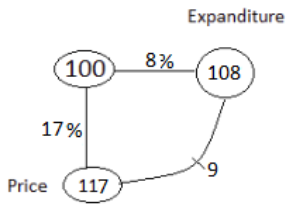Desired percentage = 9/117 x 100 ≈ 7.7%

Sol 16. (a)

We know that,

Price x Consumption = Expenditure

21% = 21100 and 12% = 325

Initial : New

Price                    100   :   121

Expenditure         25    :    28

_______________

Consumption    25/100  :  28/121

121      :   112

%age decrease in consumption = 121-112/121 x 100 ≈ 7.4% ans

Alternate :

Direct Trick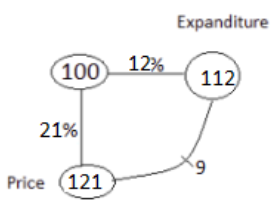Desired percentage = 9/121 x 100≈ 7.4%

Sol 17. (c)

We know that,

Price x Consumption = Expenditure

17% = 17100 and 7% = 7100

Initial : New

Price                     100   :   117

Expenditure         100    :  107

_______________

Consumption    100/100  :  107/117

117      :   107

%age decrease in consumption = 117-107/117  x 100 ≈ 8.5% ans

Alternate :

Direct Trick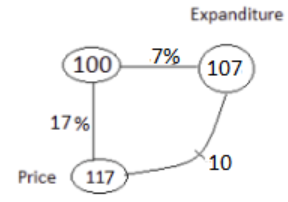Desired percentage = 10/117 x 100 ≈ 8.5%

Sol 18. (a)

We know that,

Price x Consumption = Expenditure

24% = 6/25 and 15% = 3/20

Initial  : New

Price                      25    :   31

Expenditure          20     :   23

_______________

Consumption    20/25   :  23/31

124   :   115

%age decrease in consumption = 124-115/124  x 100 ≈ 7.3% ans

Alternate :

Direct Trick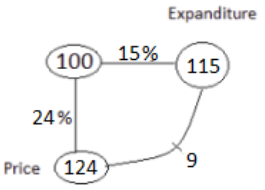Desired percentage = 9/124 x 100 ≈ 7.3%

Sol 19. (c)

We know that,

Price x Consumption = Expenditure

24% = 6/25 and 18% = 9/50

Initial  : New

Price                      25    :   31

Expenditure          50     :   59

______________

Consumption    50/25   :  59/31

62     :   59

%age decrease in consumption = 62-59/62  x 100 ≈ 4.8% ans

Alternate :

Direct Trick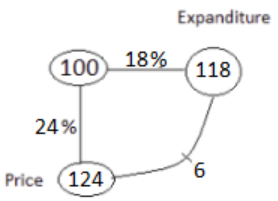Desired percentage = 6/124 x 100 ≈ 4.8%

Sol 20. (b)

We know that,

Price x Consumption = Expenditure

28% = 725 and 22% = 1150

Initial  : New

Price                      25    :   32

Expenditure          50     :   61

_______________

Consumption    50/25   :  61/32

64     :   61

%age decrease in consumption = 64-61/64 x 100 ≈ 4.7% ans

Alternate :

Direct Trick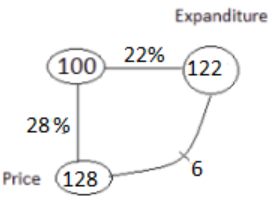Desired percentage = 6/128 x 100 ≈  4.7%

Sol 21. (a)

We know that,

Price x Consumption = Expenditure

17% = 17/100 and 5% = 1/20

Initial  : New

Price                      100  :  117

Expenditure          20     :   21

______________

Consumption    20/100 :  21/117

117   :   105

%age decrease in consumption = 117-105/117 x 100 ≈ 10.3% ans

Alternate :

Direct Trick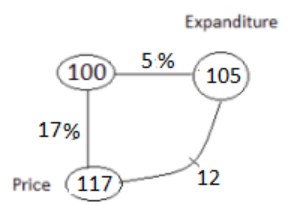Desired percentage = 12/117 x 100 ≈ 10.3%